Note: The other languages of the website are Google-translated. Back to English

## Excel 从文本字符串中删除字符、单词、数字

### 从文本字符串的左侧、右侧或中间删除字符

#### 1.1 去除文本字符串的前n个字符

###### 通过使用公式

REPLACE 函数删除前 N 个字符：

=REPLACE(string, 1, num_chars, "")
• 绳子: 要从中删除字符的文本字符串；
• 数字字符: 要删除的字符数。

=REPLACE(A4, 1, 2, "")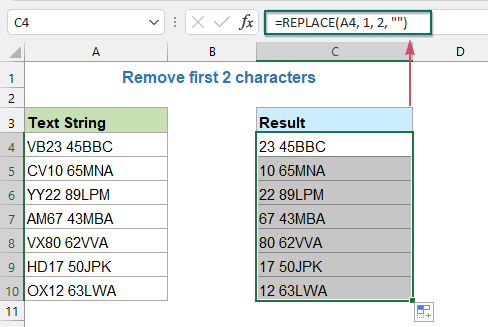RIGHT 和 LEN 函数删除前 N 个字符：

=RIGHT(string, LEN(string) - num_chars)
• 绳子: 要从中删除字符的文本字符串；
• 数字字符: 要删除的字符数。

=RIGHT(A4,LEN(A4)-2)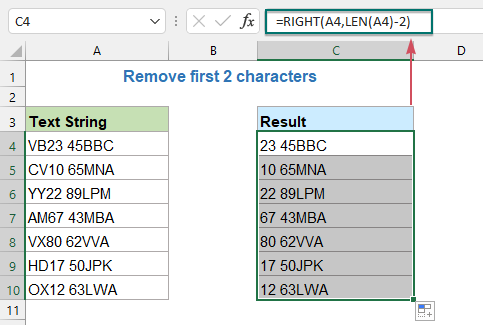###### 通过使用用户定义函数

1。 按住 Alt + F11键 键打开 Microsoft Visual Basic应用程序 窗口。

2。 点击 插页 > 模块，然后将以下代码粘贴到“模块窗口”中。

VBA 代码：从文本字符串中删除前 n 个字符

``````Function removeFirstx(rng As String, cnt As Long)
'Updateby Extendoffice
removeFirstx = Right(rng, Len(rng) - cnt)
End Function
``````

3。 然后回到工作表，然后输入以下公式： = removefirstx（A4,2） 放入空白单元格，然后向下拖动填充手柄以根据需要获取结果，请参见屏幕截图：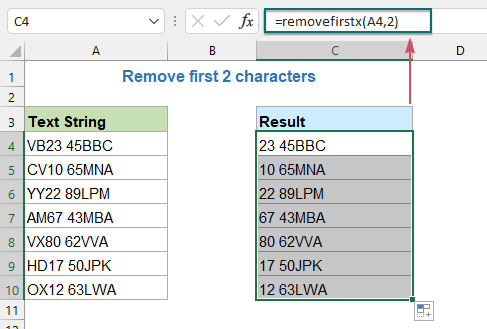#### 1.2 从文本字符串中删除最后 n 个字符

###### 通过使用公式

LEFT 和 LEN 函数删除最后 N 个字符：

=LEFT(string, LEN(string) - num_chars)
• 绳子: 要从中删除字符的文本字符串；
• 数字字符: 要删除的字符数。

=LEFT(A4, LEN(A4) - 3)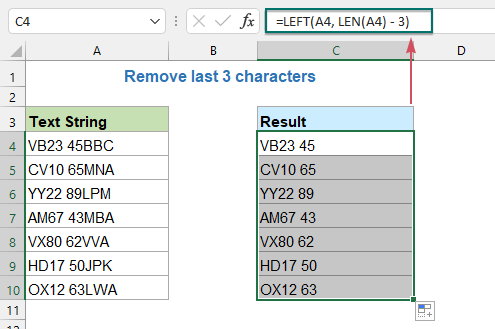###### 通过使用用户定义函数

1。 按住 Alt + F11键 键打开 Microsoft Visual Basic应用程序 窗口。

2。 点击 插页 > 模块，然后将以下代码粘贴到“模块窗口”中。

VBA 代码：从文本字符串中删除最后 n 个字符

``````Function removeLastx(rng As String, cnt As Long)
'Updateby Extendoffice
removeLastx = Left(rng, Len(rng) - cnt)
End Function
``````

3. 然后返回到工作表，并输入以下公式： = removelastx（A4,3） 放入空白单元格，然后向下拖动填充手柄以根据需要获取结果，请参见屏幕截图：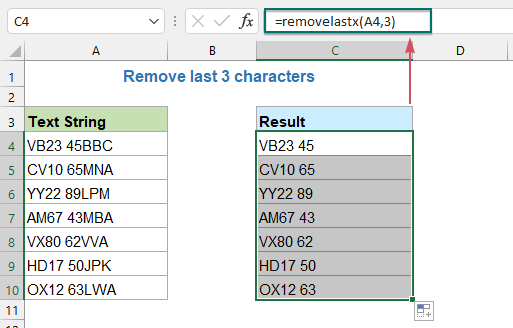#### 1.3 使用强大的功能去除首尾n个字符或某个位置的字符

1. 选择要删除字符的单元格，然后单击 库工具 > 文本 > 按位置删除，请参见屏幕截图：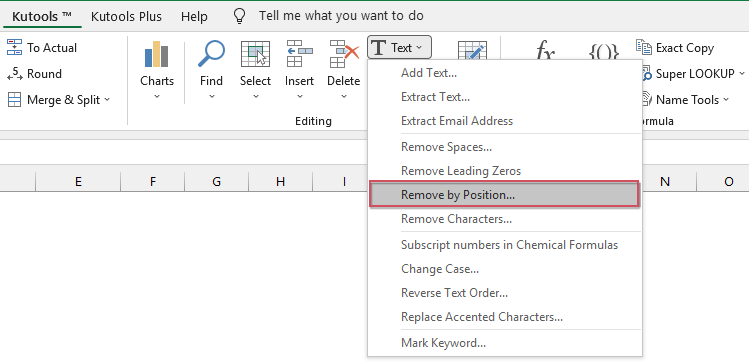2。 在 按位置删除 对话框，请执行以下操作：

2.1 从单元格中删除前 n 个字符：

• In 数值 文本框中，键入要从字符串中删除的字符数。 在这个例子中，我将删除前 2 个字符。
• 选择 从左边 选项 职务 部分。
• 然后，单击 Ok or 申请 按钮以获得如下图所示的结果。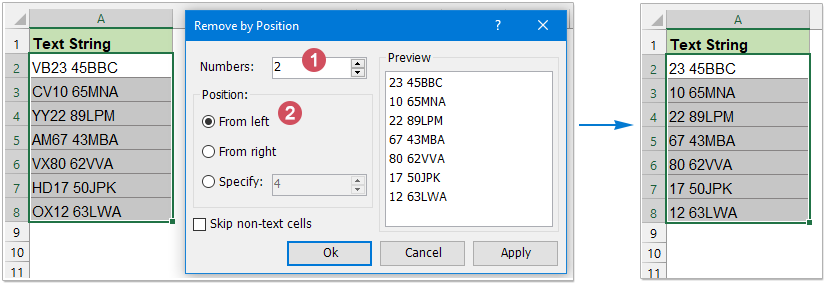2.2 从单元格中删除最后 n 个字符：

• In 数值 文本框中，键入要从字符串中删除的字符数。 在这个例子中，我将删除最后 3 个字符。
• 选择 从右边 选项 职务 部分。
• 然后，单击 Ok or 申请 按钮以获得如下图所示的结果。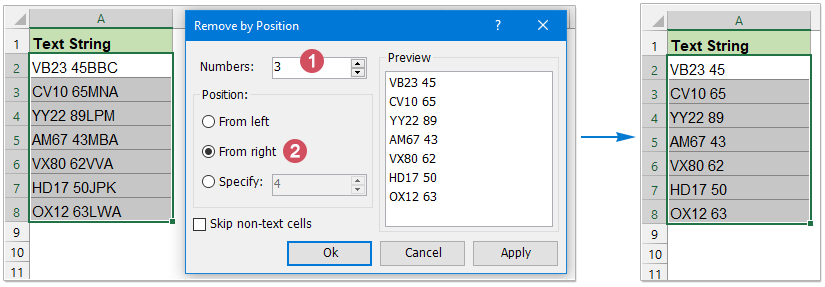2.3 去除单元格特定位置的n个字符：

• In 数值 文本框中，键入要从字符串中删除的字符数。 在这个例子中，我将从某个位置删除 3 个字符。
• 选择 指定 选项，然后在文本框中键入要从其开始删除字符的数字 职务 部分。 在这里，我将从第三个字符中删除字符。
• 然后，单击 Ok or 申请 按钮以获得如下图所示的结果。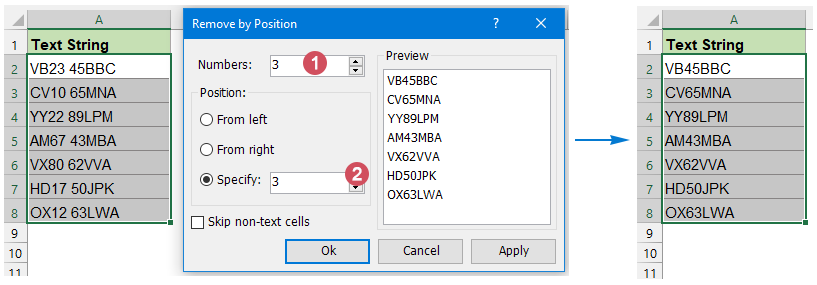#### 1.4 用公式去除文本字符串的前n个和后n个字符

=MID(string, left_chars + 1, LEN(string) - (left_chars + right_chars)
• 绳子: 要从中删除字符的文本字符串；
• left_chars: 从左边移除的字符数；
• right_chars: 从右边删除的字符数。

=MID(A4, 7+1, LEN(A4) - (7+5))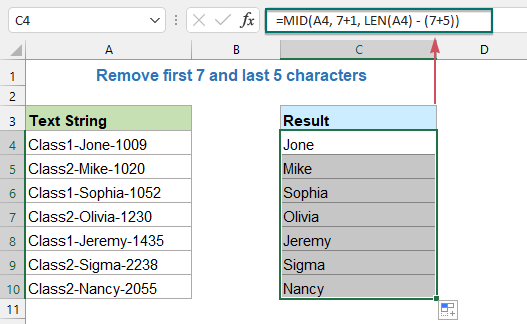### 从文本字符串中删除不需要的/特殊字符

#### 2.1 去除文本字符串中的一些特殊字符

###### 使用 SUBSTITUTE 函数从文本字符串中删除几个特殊字符

=SUBSTITUTE(SUBSTITUTE(SUBSTITUTE(string_cell, char1, ""), char2, ""), char3, "")
• 字符串单元格：单元格包含要从中删除特殊字符的文本字符串；
• 字符 1、字符 2、字符 3：要删除的不需要的字符。

=SUBSTITUTE(SUBSTITUTE(SUBSTITUTE(SUBSTITUTE(A2, "#", ""), "&", ""), "*", ""), "%", "")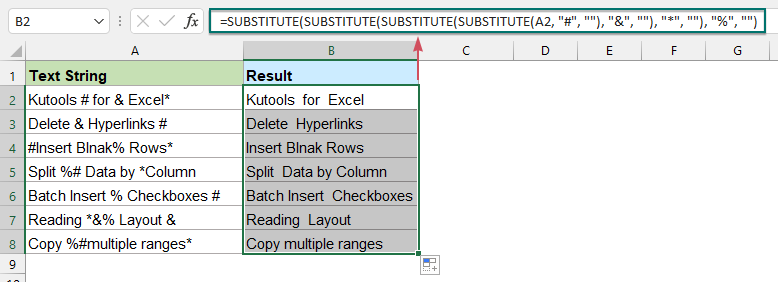###### 使用用户定义函数从文本字符串中删除多个特殊字符

1。 按住 Alt + F11键 键打开 Microsoft Visual Basic应用程序 窗口。

2。 点击 插页 > 模块，并将以下代码粘贴到模块窗口中。

VBA 代码：从文本字符串中删除多个特殊字符

``````Function RemoveUnwantedChars(Str As String, xchars As String)
'Updateby Extendoffice
For Index = 1 To Len(xchars)
Str = Replace(Str, Mid(xchars, Index, 1), "")
Next
RemoveUnwantedChars = Str
End Function
``````

3. 然后，关闭代码窗口并返回工作表，输入此公式 =删除不需要的字符（A2，\$D\$2） 到一个空白单元格中输出结果，然后向下拖动填充手柄以根据需要获取结果，请参见屏幕截图：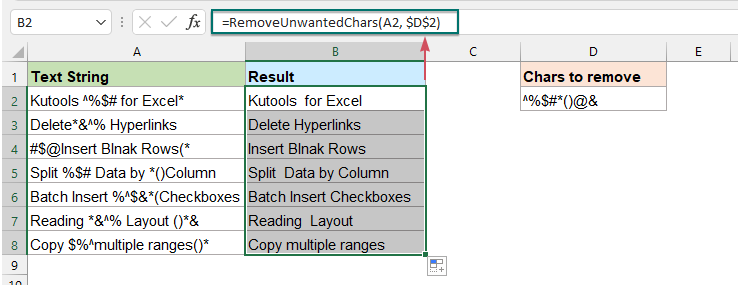###### 从具有惊人功能的文本字符串中删除多个特殊字符

1. 选择要从中删除特殊字符的单元格范围，然后单击 库工具 > 文本 > 删除字符，请参见屏幕截图：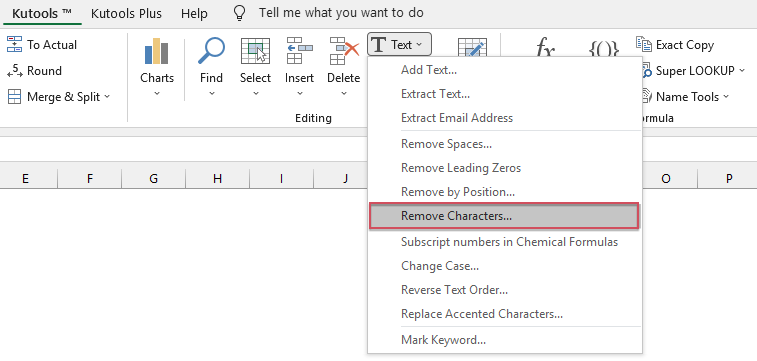2。 在 删除字符 对话框：

• 单向阀 定制 选项下 删除字符 部分。
• 然后在要删除的文本框中输入特殊字符。
• 然后，单击 Ok or 申请 按钮一次删除您指定的字符。 看截图：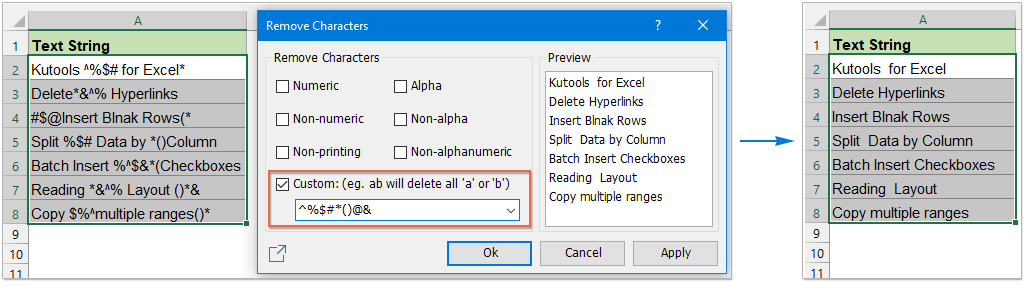#### 2.2 从文本字符串中删除所有数字

###### 使用 SUBSTITUTE 函数从文本字符串中删除数字

=SUBSTITUTE(SUBSTITUTE(SUBSTITUTE(SUBSTITUTE(SUBSTITUTE(SUBSTITUTE(SUBSTITUTE(SUBSTITUTE(SUBSTITUTE(SUBSTITUTE(A2,1,""),2,""),3,""),4,""),5,""),6,""),7,""),8,""),9,""),0,"")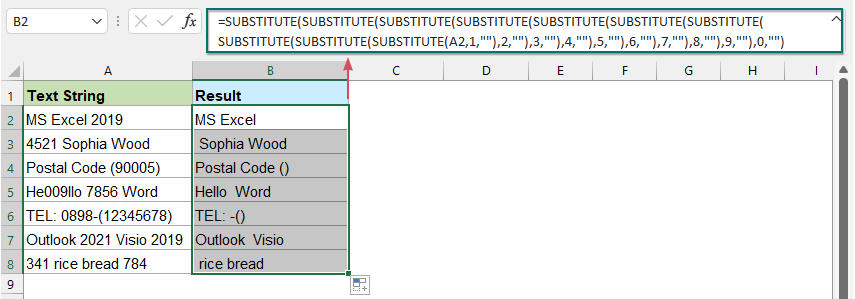###### 使用 TEXTJOIN 函数从文本字符串中删除数字

=TEXTJOIN("", TRUE, IF(ISERR(MID(A2, ROW(INDIRECT( "1:"&LEN(A2) )), 1) *1), MID(A2, ROW(INDIRECT("1:"&LEN(A2))), 1), ""))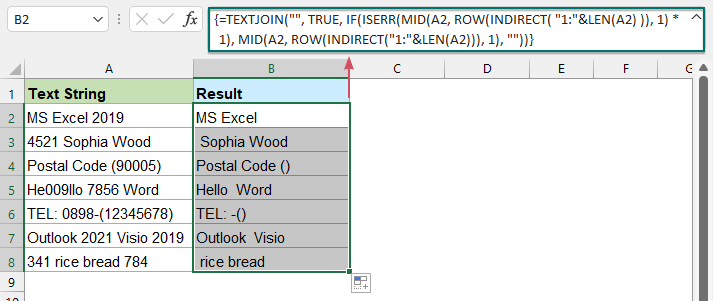###### 使用用户定义函数从文本字符串中删除数字

1。 按住 Alt + F11键 键打开 Microsoft Visual Basic应用程序 窗口。

2。 点击 插页 > 模块，并将以下代码粘贴到模块窗口中。

VBA 代码：从文本字符串中删除数字

``````Function RemoveNumbers(Txt As String) As String
'Updateby Extendoffice
With CreateObject("VBScript.RegExp")
.Global = True
.Pattern = "[0-9]"
RemoveNumbers = .Replace(Txt, "")
End With
End Function
``````

3. 然后，关闭并退出代码窗口，返回工作表，输入以下公式： =删除号码（A2） 进入一个空白单元格，然后将填充手柄向下拖动到要应用此公式的单元格，请参见屏幕截图：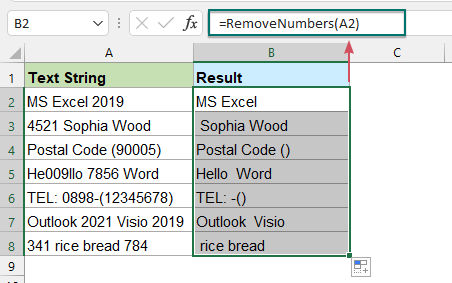###### 使用方便的选项从文本字符串中删除数字

1. 选择要删除数字的单元格范围，然后单击 库工具 > 文本 > 删除字符.

2。 在 删除字符 对话框，请执行以下操作：

• 单向阀 数字 选项下 删除字符 部分。
• 然后，单击 Ok or 申请 按钮立即删除数字。 看截图：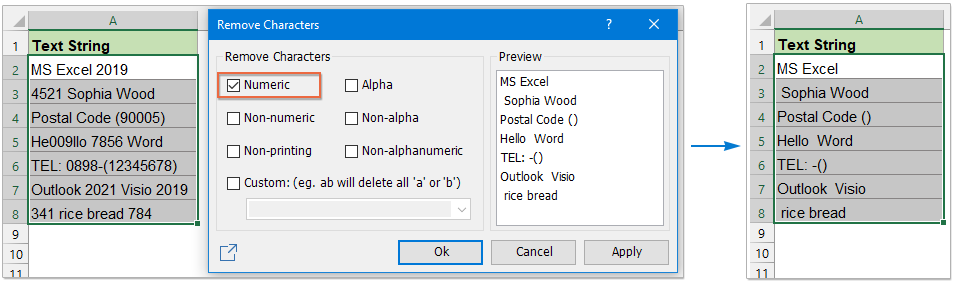#### 2.3 去除文本字符串中的非数字字符

###### 在 Excel 2016 及更早版本中使用公式从文本字符串中删除非数字字符

=SUMPRODUCT(MID(0&A2, LARGE(INDEX(ISNUMBER(--MID(A2, ROW(INDIRECT("1:"&LEN(A2))), 1)) * ROW(INDIRECT("1:"&LEN(A2))), 0), ROW(INDIRECT("1:"&LEN(A2))))+1, 1) * 10^ROW(INDIRECT("1:"&LEN(A2)))/10)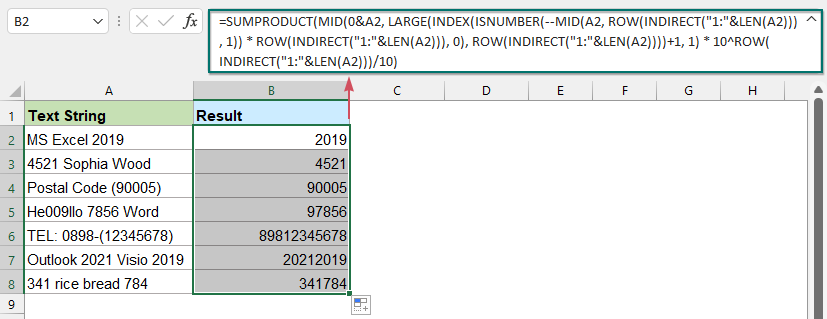###### 使用 Excel 2019、2021、365 中的 TEXTJOIN 函数从文本字符串中删除非数字字符

=TEXTJOIN("",TRUE,IFERROR(MID(A2,ROW(INDIRECT("1:100")),1)+0,""))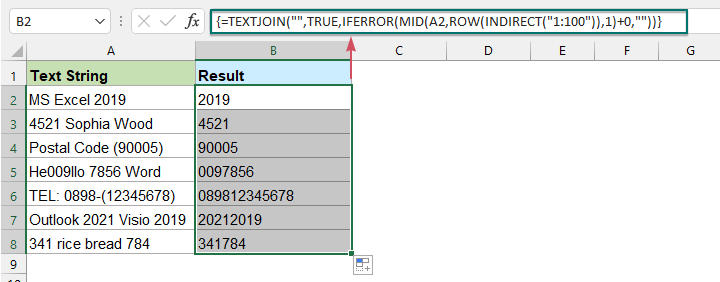###### 使用用户定义函数从文本字符串中删除非数字字符

1。 按住 Alt + F11键 键打开 Microsoft Visual Basic应用程序 窗口。

2。 点击 插页 > 模块，并将以下代码粘贴到模块窗口中。

VBA 代码：从文本字符串中删除非数字字符

``````Function Removenonnumeric(str As String) As String
'Updateby Extendoffice
With CreateObject("VBScript.RegExp")
.Global = True
.Pattern = "[^0-9]"
Removenonnumeric = .Replace(str, "")
End With
End Function
``````

3. 然后，关闭并退出代码窗口，返回工作表，输入以下公式： =移除非数字(A2) 进入一个空白单元格，然后将填充手柄向下拖动到要应用此公式的单元格，只有数字将被提取，如下图所示：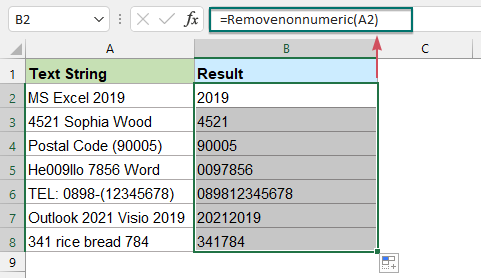###### 使用简单的功能从文本字符串中删除非数字字符

1. 选择要从中删除非数字字符的单元格范围，然后单击 库工具 > 文本 > 删除字符.

2。 在 删除字符 对话框中，请设置以下操作：

• 单向阀 非数值 选项下 删除字符 部分。
• 然后，单击 Ok or 申请 按钮立即删除所有非数字字符。 看截图：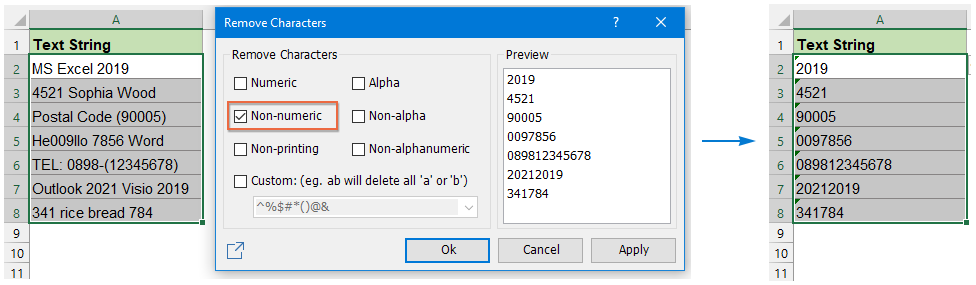#### 2.4 将一个单元格中的文本和数字分成两列

###### 使用用户定义函数将一个单元格中的文本和数字分成两列

1。 按住 Alt + F11键 键打开 Microsoft Visual Basic应用程序 窗口。

2。 点击 插页 > 模块，并将以下代码粘贴到模块窗口中。

VBA代码：将文本字符串中的文本和数字分成两列

``````Function SplitText(pWorkRng As Range, pIsNumber As Boolean) As String
'Updateby Extendoffice
Dim xLen As Long
Dim xStr As String
xLen = VBA.Len(pWorkRng.Value)
For i = 1 To xLen
xStr = VBA.Mid(pWorkRng.Value, i, 1)
If ((VBA.IsNumeric(xStr) And pIsNumber) Or (Not (VBA.IsNumeric(xStr)) And Not (pIsNumber))) Then
SplitText = SplitText + xStr
End If
Next
End Function
``````

3. 然后，关闭并退出代码窗口，返回工作表，输入以下公式： = SplitText（A2，FALSE） 进入一个空白单元格，然后将填充手柄向下拖动到要填充此公式的单元格以获取所有文本，请参见屏幕截图：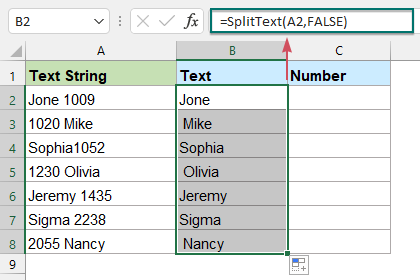4. 然后，继续输入这个公式： = SplitText（A2，TRUE） 到另一个单元格中，然后将填充手柄向下拖动到要填充此公式以获取数字的单元格，请参见屏幕截图：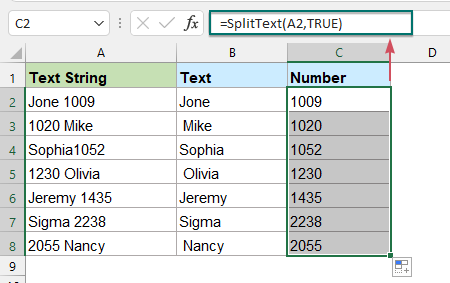###### 使用简单的功能将一个单元格中的文本和数字分成两列

1. 选择要拆分的单元格范围，然后单击 库工具 > 合并与拆分 > 分裂细胞，请参见屏幕截图：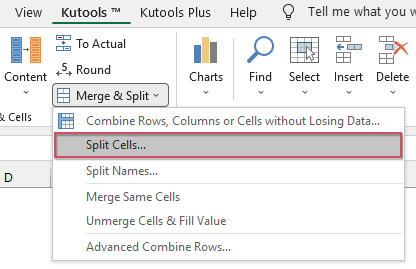2。 在 分裂细胞 对话框中选择 拆分为列 选项下 类型 部分，然后检查 文字和数字 来自 分割为 部分，请参见屏幕截图：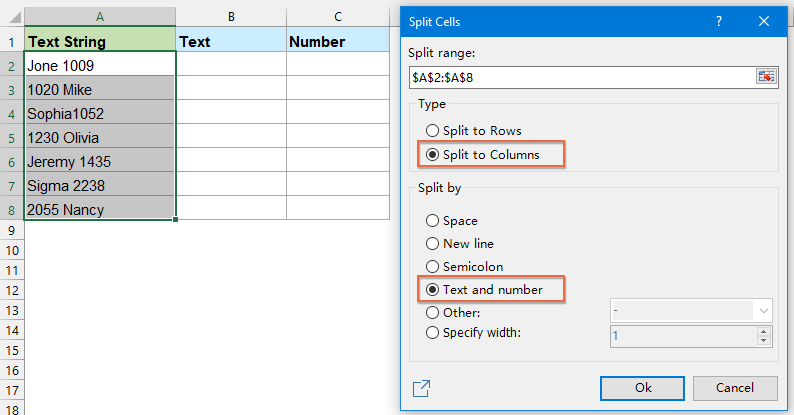3。 然后，点击 Ok 按钮，另一个 分裂细胞 弹出对话框，选择一个单元格输出分隔的文本和数字，然后点击 OK 按钮。 现在，您可以看到所选单元格中的文本和数字一次分为两列，如下图所示：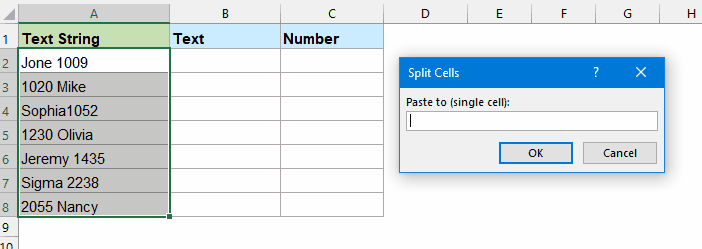#### 2.5 去除文本字符串中的换行符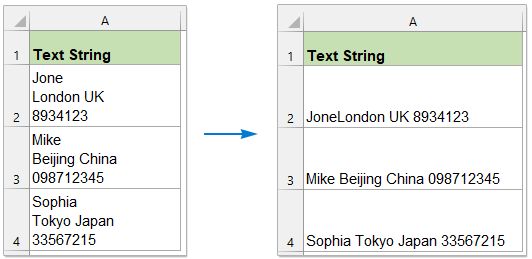###### 使用查找和替换功能从文本字符串中删除换行符

1. 选择要从中删除换行符的数据范围。

2。 然后，点击 主页 > 查找和选择 > 更换 （或按 Ctrl + H 键）去 查找和替换 对话框，请参见屏幕截图：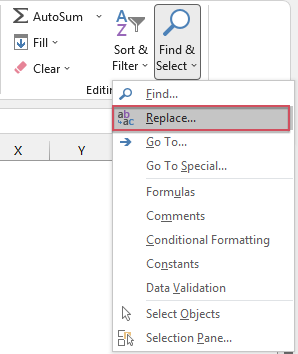3。 在弹出 查找和替换 对话框，请执行以下操作：

• 将光标放在 查找内容 场和新闻 Ctrl + J 在键盘上，您可能看不到任何内容，但已插入换行符。
• 更换 字段，将此字段留空以删除换行符或按 太空霸r 一次用空格替换换行符。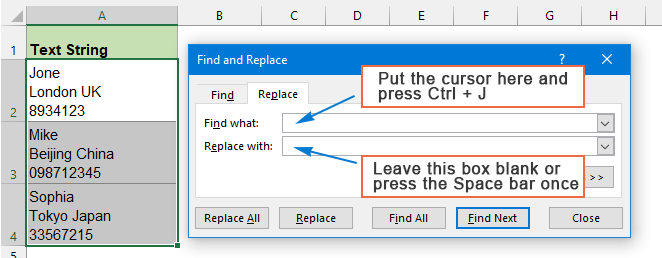4。 然后，点击 “全部替换” 按钮，所选单元格中的所有换行符将立即被删除或替换为空格。 看截图：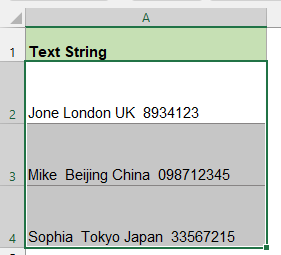###### 使用 SUBSTITUTE 函数从文本字符串中删除换行符

=SUBSTITUTE(A2,CHAR(10),"")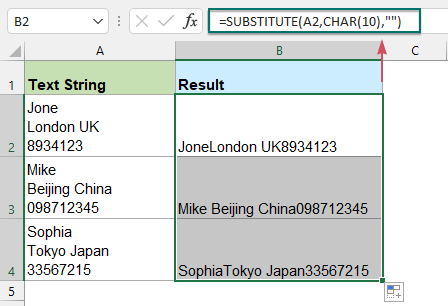=SUBSTITUTE(A2,CHAR(10),", ")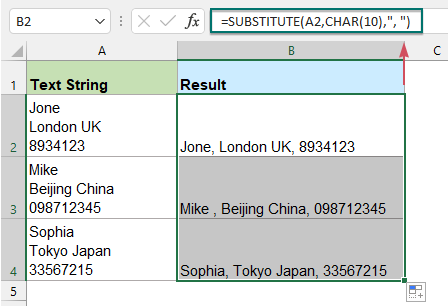###### 使用 VBA 代码从文本字符串中删除换行符

1。 按住 Alt + F11键 键打开 Microsoft Visual Basic应用程序 窗口。

2。 点击 插页 > 模块，并将以下代码粘贴到模块窗口中。

VBA 代码：从文本字符串中删除换行符

``````Sub RemoveCarriage()
'Updateby Extendoffice
Dim Rng As Range
Dim WorkRng As Range
On Error Resume Next
xTitleId = "KutoolsforExcel"
Set WorkRng = Application.Selection
Set WorkRng = Application.InputBox("Range", xTitleId, WorkRng.Address, Type:=8)
For Each Rng In WorkRng
Rng.Value = Replace(Rng.Value, Chr(10), "")
Next
End Sub
``````

3。 然后按 F5 键运行这段代码，会出现一个提示框，选择要去掉换行符的范围，看截图：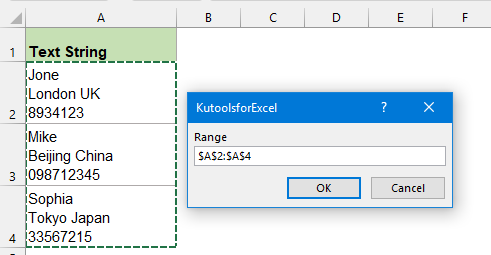4。 然后，单击 OK 按钮，所有换行符将从所选数据范围中删除。

###### 使用智能选项从文本字符串中删除换行符

1. 选择要删除换行符的单元格范围，然后单击 库工具 > 文本 > 删除字符.

2。 在 删除字符 对话框中，请设置以下操作：

• 单向阀 非印刷 选项下 删除字符 部分。
• 然后，单击 Ok or 申请 按钮从选定的数据范围中删除所有换行符。 看截图：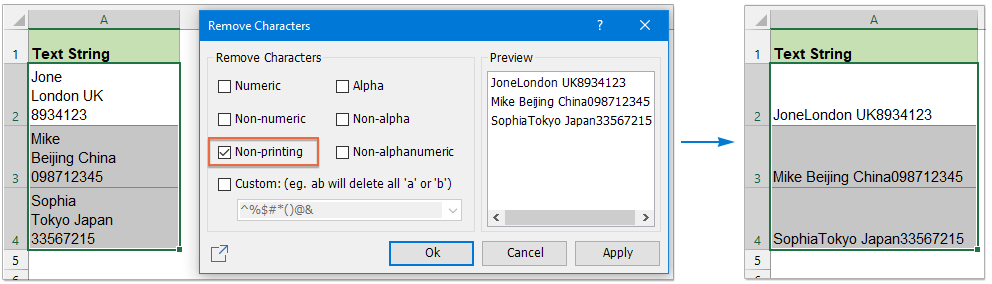#### 2.6 从文本字符串中删除空格（前导、尾随、额外或所有空格）

###### 使用 TRIM 函数从文本字符串中删除多余的空格（前导、尾随、多余）

=TRIM(A2)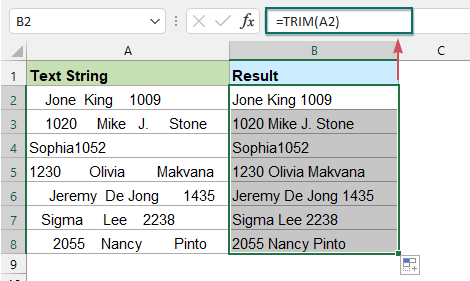###### 从文本字符串中删除所有空格

=SUBSTITUTE(A2," ","")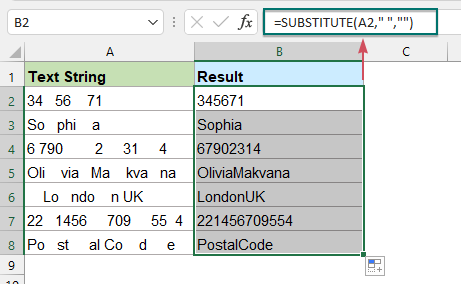1. 选择要从中删除所有空格的数据范围。

2。 然后，点击 主页 > 查找和选择 > 更换 （或按 Ctrl + H 键）转到 查找和替换 对话框，在打开的 查找和替换 对话框，请执行以下操作：

• 新闻 空格键 查找内容 领域;
• 更换 字段，将此字段留空。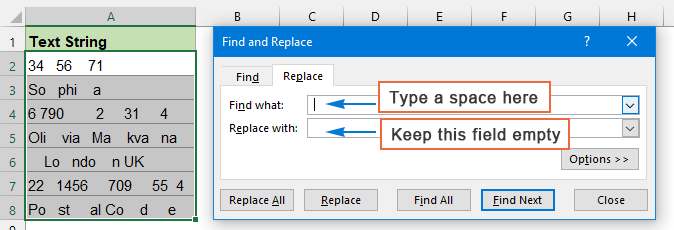3。 然后，单击 “全部替换” 按钮，将立即删除所选单元格中的所有空格。 看截图：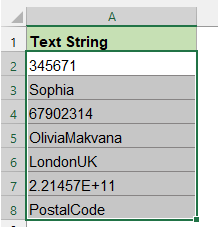###### 用强大的功能去除文本字符串中的各种空格

Kutools for Excel 有一个强大的功能—— 删除空间, 使用此实用程序，您不仅可以在一个对话框中删除前导空格、尾随空格、多余空格，还可以删除选定范围内的所有空格，这将提高您的工作效率。

1. 选择要从中删除空格的数据范围，然后单击 库工具 > 文本 > 删除空间。 看截图：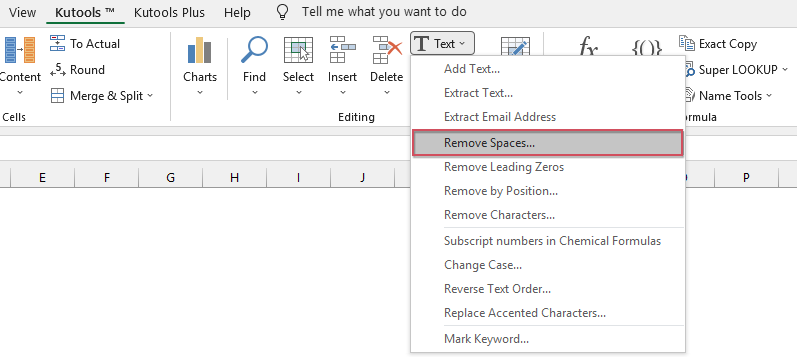2。 在 删除空间 对话框中，选择一种要从 空间类型:

• 去除前导空格，请选择 前导空间 选项;
• 删除尾随空格，请选择 尾随空格 选项;
• 一次删除前导空格和尾随空格，请选择 前导和尾随空格 选项;
• 删除所有多余的空格，请选择 所有多余的空间 选项;
• 删除所有空格，请选择 所有空间 选项。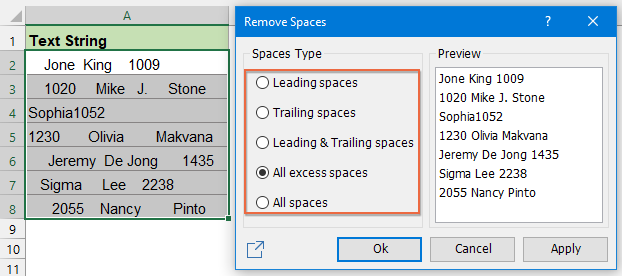3。 然后，点击 Ok or 申请 按钮，您将获得所需的结果。

### 删除特定字符之前或之后的字符/文本

#### 3.1 删除第一个特定字符之前或之后的文本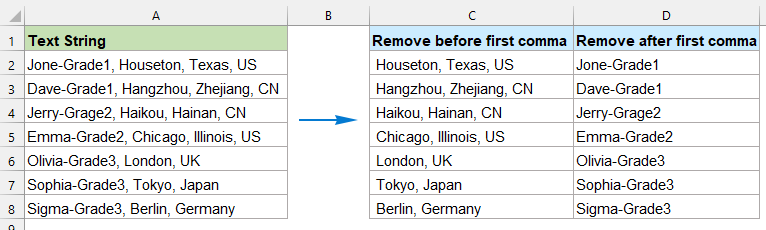###### 使用公式删除第一个特定字符之前的文本

=RIGHT(cell, LEN(cell)-FIND("char", cell))
• 细胞：要从中删除文本的单元格引用或文本字符串；
• 坦克：您要基于其删除文本的特定分隔符。

=RIGHT(A2,LEN(A2)-FIND(",",A2))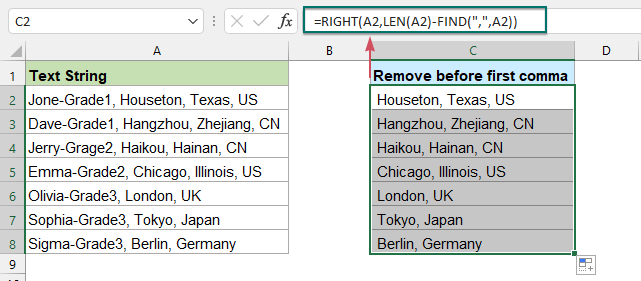###### 使用公式删除第一个特定字符后的文本

=LEFT(cell,FIND("char",cell)-1)
• 细胞：要从中删除文本的单元格引用或文本字符串；
• 坦克：您要基于其删除文本的特定分隔符。

=LEFT(A2,FIND(",",A2)-1)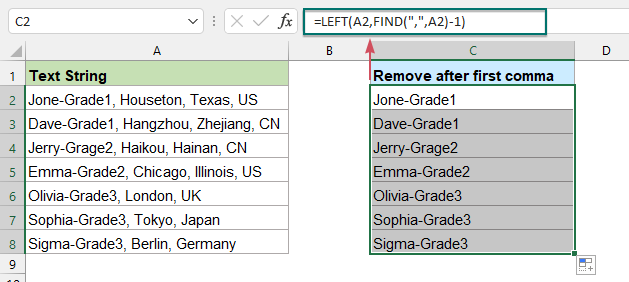#### 3.2 删除第 N 次出现之前或之后的文本

###### 使用公式删除第 N 个字符之前的文本

=RIGHT(cell,LEN(cell)-FIND("#",SUBSTITUTE(cell,"char","#",N)))
• 细胞：要从中删除文本的单元格引用或文本字符串；
• 坦克：您要根据其删除文本的特定分隔符；
• N: 要删除文本之前的字符出现。

=RIGHT(A2,LEN(A2)-FIND("#",SUBSTITUTE(A2,",","#",2)))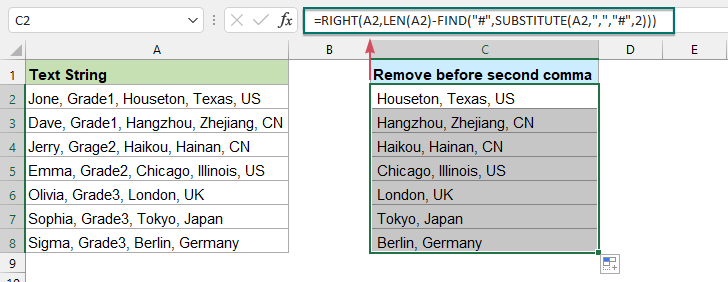###### 使用公式删除第 N 次出现的字符后的文本

=LEFT(cell, FIND("#", SUBSTITUTE(cell, "char", "#", N)) -1)
• 细胞：要从中删除文本的单元格引用或文本字符串；
• 坦克：您要根据其删除文本的特定分隔符；
• N: 要删除文本的字符出现位置。

=LEFT(A2, FIND("#", SUBSTITUTE(A2, ",", "#", 2)) -1)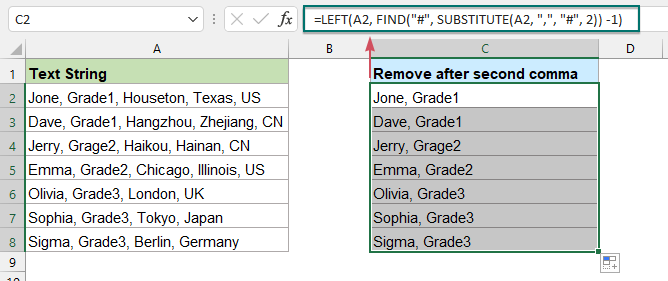###### 使用用户定义函数删除第 N 次出现之前或之后的文本

1。 按住 Alt + F11键 键打开 Microsoft Visual Basic应用程序 窗口。

2。 点击 插页 > 模块，并将以下代码粘贴到模块窗口中。

VBA 代码：删除第 N 次出现字符之前或之后的文本

``````Function RemoveTextOccurrence(Str As String, Delimiter As String, Occurrence As Integer, IsAfter As Boolean)
Dim xStr As String
Dim xStrLen, xF, xIntStart As Integer
xStr = Str
xStrLen = Len(xStr)
xIntStart = 1
For xF = 1 To Occurrence
xIntStart = InStr(xIntStart + 1, xStr, Delimiter, vbTextCompare)
If (xIntStart = 0) Or (xIntStart < 0) Then
If IsAfter Then
RemoveTextOccurrence = xStr
Else
RemoveTextOccurrence = ""
End If
Exit Function
End If
Next
If IsAfter Then
RemoveTextOccurrence = Mid(Str, 1, xIntStart - 1)
Else
RemoveTextOccurrence = Mid(Str, xIntStart + 1)
End If
End Function
``````

3. 然后，关闭并退出代码窗口，返回工作表，使用以下公式：

=RemoveTextOccurrence(A2, ", ", 2, FALSE)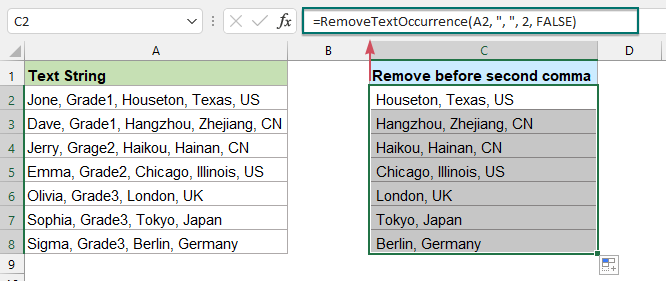=RemoveTextOccurrence(A2, ", ", 2, TRUE)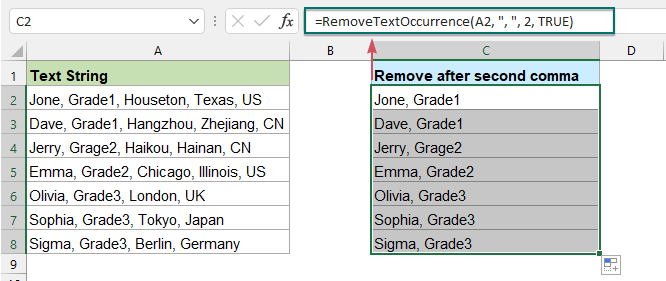#### 3.3 删除最后一次出现之前或之后的文本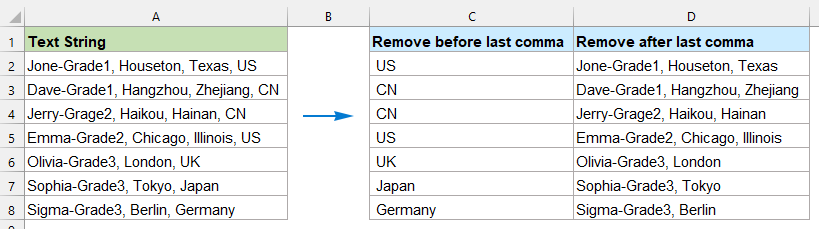###### 使用公式删除最后一次出现字符之前的文本

=RIGHT(cell,LEN(cell)-SEARCH("#",SUBSTITUTE(cell,"char","#",LEN(cell)-LEN(SUBSTITUTE(cell,"char","")))))
• 细胞：要从中删除文本的单元格引用或文本字符串；
• 坦克：您要根据其删除文本的特定分隔符；

=RIGHT(A2,LEN(A2)-SEARCH("#",SUBSTITUTE(A2,",","#",LEN(A2)-LEN(SUBSTITUTE(A2,",","")))))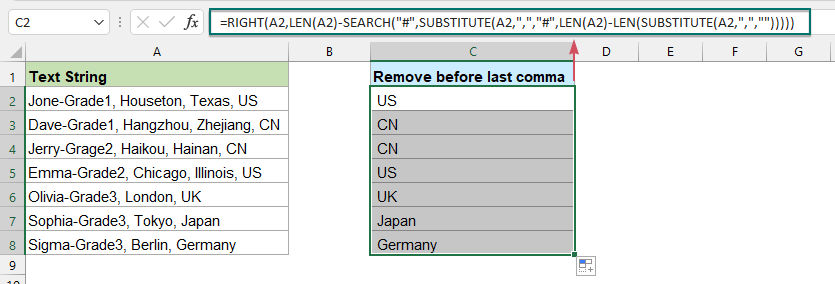###### 在最后一次出现带有公式的字符后删除文本

=LEFT(cell,FIND("#",SUBSTITUTE(cell,"char","#",LEN(cell)-LEN(SUBSTITUTE(cell,"char",""))))-1)
• 细胞：要从中删除文本的单元格引用或文本字符串；
• 坦克：您要根据其删除文本的特定分隔符；

=LEFT(A2,FIND("#",SUBSTITUTE(A2,",","#",LEN(A2)-LEN(SUBSTITUTE(A2,",",""))))-1)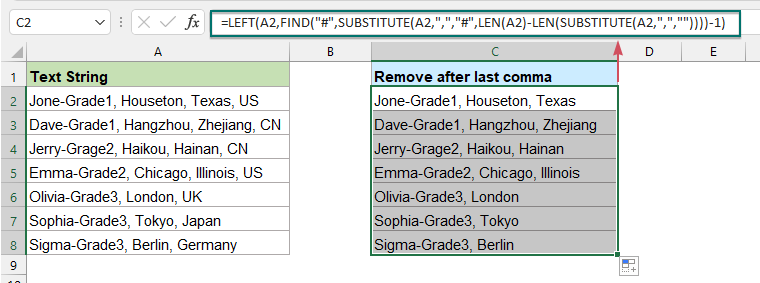#### 3.4 去掉括号之间的文字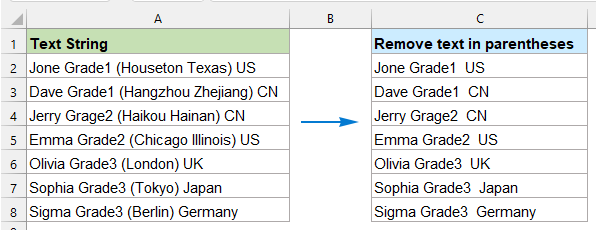###### 使用查找和替换功能删除括号之间的文本

1. 选择要删除括号之间文本的数据列表。

2。 然后，点击 主页 > 查找和选择 > 更换 （或按 Ctrl + H 键）转到查找和替换对话框，在 查找和替换 对话框中，进行以下操作：

• 查找内容 字段中，键入 （*） 进入文本框；
• 更换 字段，将此字段留空。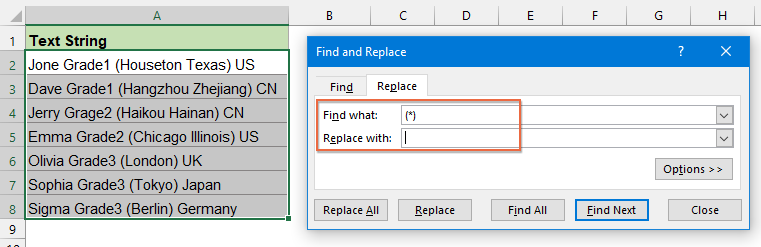3。 然后，点击 “全部替换” 按钮，选中单元格中括号内的所有字符（包括括号）将被一次性删除。 看截图：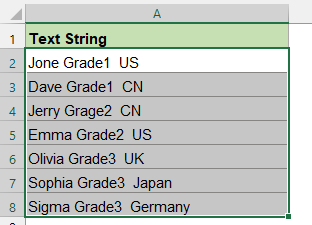###### 用公式删除括号之间的文本

=SUBSTITUTE(text,MID(LEFT(text,FIND(")",text)),FIND("(",text),LEN(text)),"")
• 文本：要从中删除字符的文本字符串或单元格引用。

=SUBSTITUTE(A2,MID(LEFT(A2,FIND(")",A2)),FIND("(",A2),LEN(A2)),"")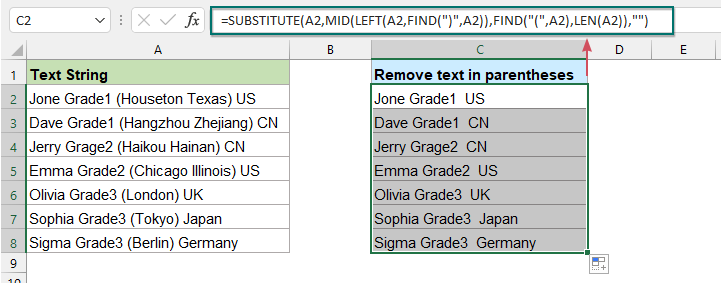=IFERROR(SUBSTITUTE(A2,MID(LEFT(A2,FIND(")",A2)),FIND("(",A2),LEN(A2)),""),A2)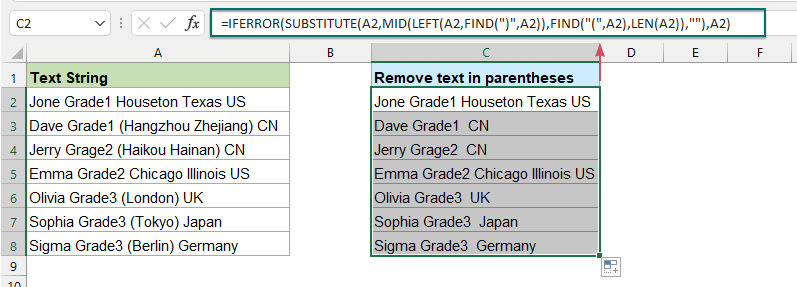###### 使用用户定义函数删除括号之间的文本

1。 按住 Alt + F11键 键打开 Microsoft Visual Basic应用程序 窗口。

2。 点击 插页 > 模块，并将以下代码粘贴到模块窗口中。

VBA 代码：删除括号之间的文本

``````Function remtxt(ByVal str As String) As String
'Updateby Extendoffice
While InStr(str, "(") > 0 And InStr(str, ")") > InStr(str, "(")
str = Left(str, InStr(str, "(") - 1) & Mid(str, InStr(str, ")") + 1)
Wend
remtxt = Trim(str)
End Function
``````

3. 然后，返回到工作表，并在空白单元格中输入此公式： = remtxt（A2），然后将填充手柄向下拖动到要应用此公式的单元格，所有括号内的所有文本（包括括号）都将被删除，如下图所示：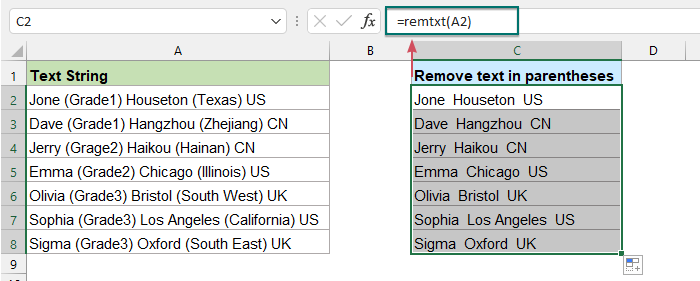### 从文本字符串中删除单词

#### 4.1 从文本字符串中删除第一个或最后一个单词

###### 使用公式从文本字符串中删除第一个单词

=RIGHT(text,LEN(text)-FIND(" ",text))
• 文本：要从中删除第一个单词的文本字符串或单元格引用。

=RIGHT(A2,LEN(A2)-FIND(" ",A2))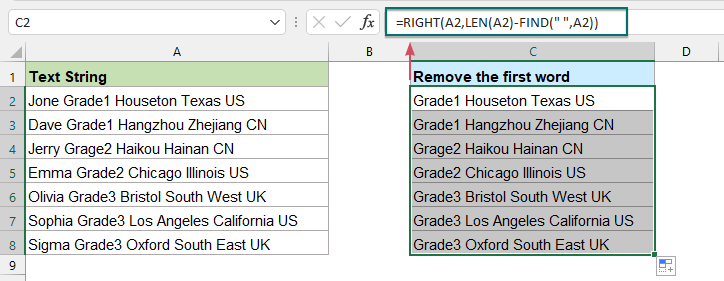=MID(TRIM(text),1+FIND("~",SUBSTITUTE(TRIM(text)," ","~",N)),255)
• 文本：要从中删除前 n 个单词的文本字符串或单元格引用；
• N: 表示要从文本字符串的开头删除多少个单词。

=MID(TRIM(A2),1+FIND("~",SUBSTITUTE(TRIM(A2)," ","~",2)),255)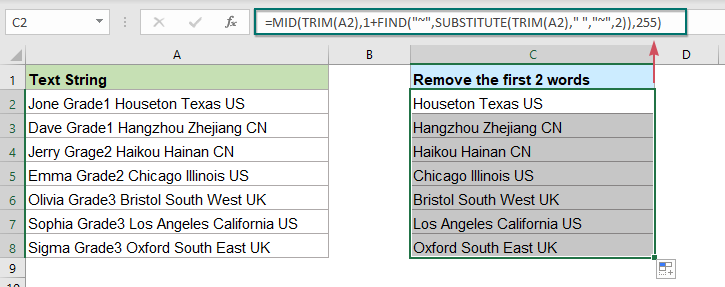###### 使用公式从文本字符串中删除最后一个单词

=LEFT(TRIM(text),FIND("~",SUBSTITUTE(text," ","~",LEN(TRIM(text))-LEN(SUBSTITUTE(TRIM(text)," ",""))))-1)
• 文本：要从中删除最后一个单词的文本字符串或单元格引用；

=LEFT(TRIM(A2),FIND("~",SUBSTITUTE(A2," ","~",LEN(TRIM(A2))-LEN(SUBSTITUTE(TRIM(A2)," ",""))))-1)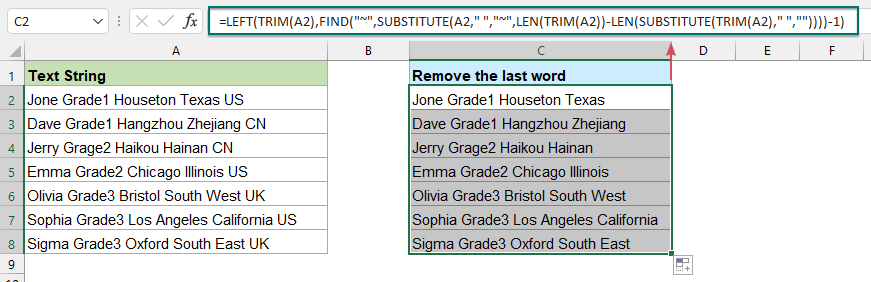=LEFT(text,FIND("~",SUBSTITUTE(text," ","~",LEN(text)-LEN(SUBSTITUTE(text," ",""))-(N-1))))
• 文本: 要从中删除最后 n 个单词的文本字符串或单元格引用；
• N：表示要从文本字符串末尾删除的单词数。

=LEFT(A2,FIND("~",SUBSTITUTE(A2," ","~",LEN(A2)-LEN(SUBSTITUTE(A2," ",""))-(3-1))))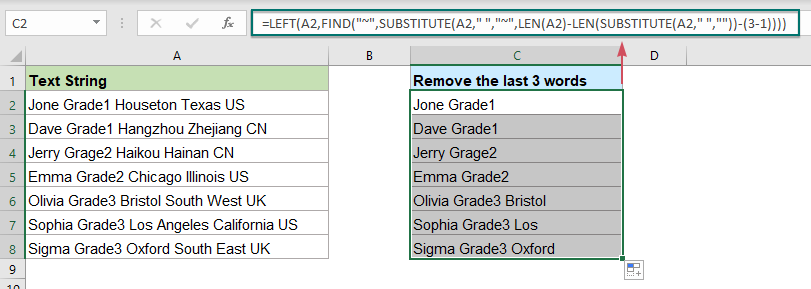#### 4.2 去除单元格内重复的字符或单词

###### 使用用户定义函数删除单元格中的重复字符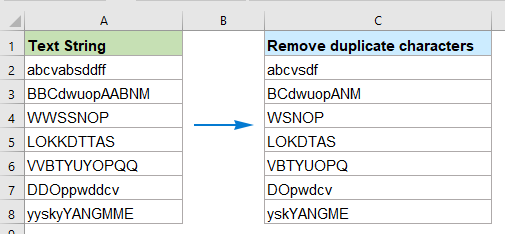1。 按住 Alt + F11键 键打开 Microsoft Visual Basic应用程序 窗口。

2。 点击 插页 > 模块，并将以下代码粘贴到模块窗口中。

VBA 代码：删除单元格中的重复字符

``````Function RemoveDupeschars(pWorkRng As Range) As String
'Updateby Extendoffice
Dim xValue As String
Dim xChar As String
Dim xOutValue As String
Set xDic = CreateObject("Scripting.Dictionary")
xValue = pWorkRng.Value
For i = 1 To VBA.Len(xValue)
xChar = VBA.Mid(xValue, i, 1)
If xDic.Exists(xChar) Then
Else
xDic(xChar) = ""
xOutValue = xOutValue & xChar
End If
Next
RemoveDupeschars = xOutValue
End Function
``````

3. 然后关闭代码窗口，回到工作表，输入这个公式 =删除Dupeschars(A2) 到您的数据旁边的空白单元格中，然后将填充手柄拖到要应用此公式的单元格上，请参阅屏幕截图：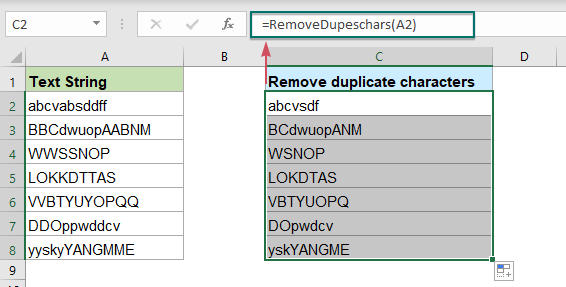###### 使用用户定义函数删除单元格中的重复单词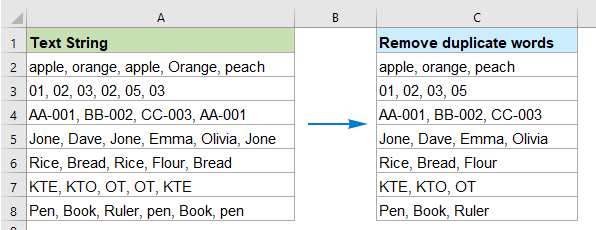1。 按住 Alt + F11键 键打开 Microsoft Visual Basic应用程序 窗口。

2。 点击 插页 > 模块，并将以下代码粘贴到模块窗口中。

VBA 代码：删除单元格中的重复单词

``````Function RemoveDupeswords(txt As String, Optional delim As String = " ") As String
'Updateby Extendoffice
Dim x
With CreateObject("Scripting.Dictionary")
.CompareMode = vbTextCompare
For Each x In Split(txt, delim)
If Trim(x) <> "" And Not .exists(Trim(x)) Then .Add Trim(x), Nothing
Next
If .Count > 0 Then RemoveDupeswords = Join(.keys, delim)
End With
End Function
``````

3. 然后关闭代码窗口，返回工作表，输入这个公式 =RemoveDupeswords(A2,",") 到您的数据旁边的空白单元格中，然后将填充手柄拖到要应用此公式的单元格上，请参阅屏幕截图：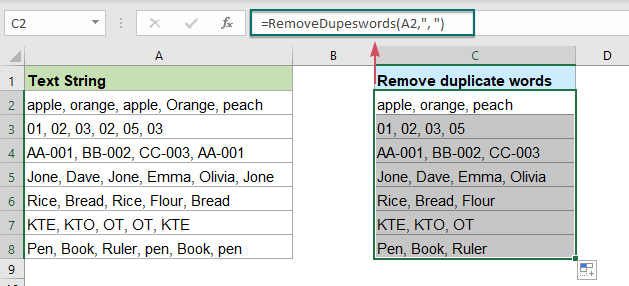#### 4.3 将文本字符串修剪为 N 个单词

###### 使用公式将文本字符串修剪为 N 个单词

=LEFT(text,FIND("~",SUBSTITUTE(text," ","~",N))-1)
• 文本：要修剪的文本字符串或单元格引用；
• N：要从给定文本字符串的左侧保留的单词数。

=LEFT(A2,FIND("~",SUBSTITUTE(A2," ","~",B2))-1)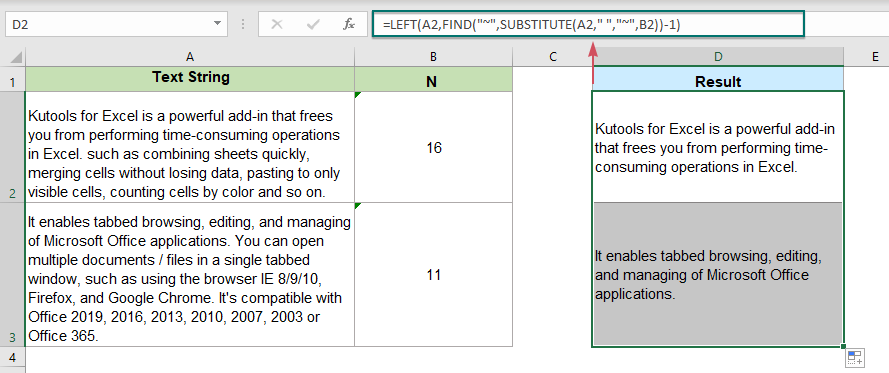###### 使用用户定义的函数将文本字符串修剪为 N 个单词

1。 按住 Alt + F11键 键打开 Microsoft Visual Basic应用程序 窗口。

2。 点击 插页 > 模块，并将以下代码粘贴到模块窗口中。

VBA 代码：将文本字符串修剪为 N 个单词

``````Function GetNWords(StrWords As String, Num_of_Words As Integer) As String
'Updateby Extendoffice
Dim xArr
Dim xRes As String
Dim xF As Integer
xStr = StrWords
If (Num_of_Words < 1) Then
GetNWords = ""
Exit Function
End If
xArr = Split(xStr, " ")
xRes = ""
On Error Resume Next
For xF = 0 To UBound(xArr)
If Trim(xArr(xF)) <> "" Then
Num_of_Words = Num_of_Words - 1
If xRes = "" Then
xRes = Trim(xArr(xF))
Else
xRes = xRes & " " & Trim(xArr(xF))
End If
End If
If Num_of_Words = 0 Then Exit For
Next
If Num_of_Words = 0 Then
GetNWords = xRes & "..."
Else
GetNWords = xRes & "..."
End If
End Function
``````

3. 然后关闭并退出代码窗口，回到工作表，输入这个公式： =GetNWords(A2,B2) 进入一个空白单元格，然后向下拖动填充手柄以将此公式应用于其他单元格，仅保留第一个特定数量的单词，如下图所示：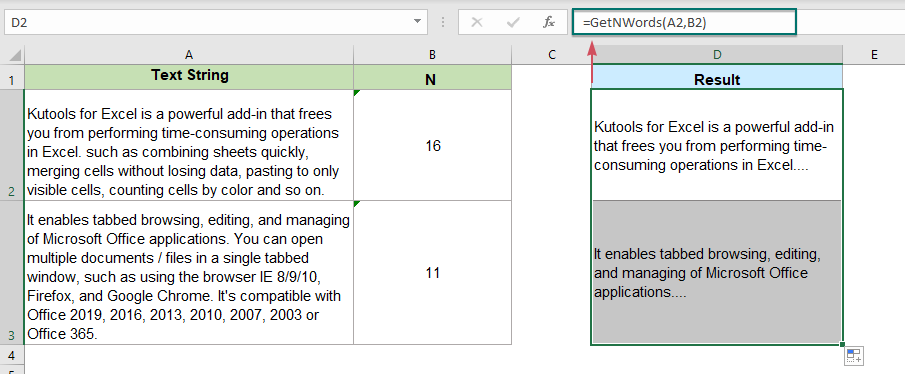## 最佳办公效率工具

### Kutools for Excel解决了您的大多数问题，并使您的生产率提高了80％

• 超级公式栏 （轻松编辑多行文本和公式）； 阅读版式 （轻松读取和编辑大量单元格）； 粘贴到过滤范围...
• 合并单元格/行/列 和保存数据； 拆分单元格内容； 合并重复的行和总和/平均值...防止细胞重复； 比较范围...
• 选择重复或唯一 行; 选择空白行 （所有单元格都是空的）； 超级查找和模糊查找 在许多工作簿中； 随机选择...
• 确切的副本 多个单元格，无需更改公式参考； 自动创建参考 到多张纸； 插入项目符号，复选框等...
• 收藏并快速插入公式，范围，图表和图片； 加密单元 带密码 创建邮件列表 并发送电子邮件...
• 提取文字，添加文本，按位置删除， 删除空间; 创建和打印分页小计； 在单元格内容和注释之间转换...
• 超级滤镜 （将过滤方案保存并应用于其他工作表）； 高级排序 按月/周/日，频率及更多； 特殊过滤器 用粗体，斜体...
• 结合工作簿和工作表; 根据关键列合并表； 将数据分割成多个工作表; 批量转换xls，xlsx和PDF...
• 数据透视表分组依据 周号，周几等 显示未锁定的单元格 用不同的颜色 突出显示具有公式/名称的单元格...### Office选项卡-将选项卡式界面引入Office，使您的工作更加轻松

• 在Word，Excel，PowerPoint中启用选项卡式编辑和阅读，发布者，Access，Visio和Project。
• 在同一窗口的新选项卡中而不是在新窗口中打开并创建多个文档。
• 每天将您的工作效率提高50％，并减少数百次鼠标单击！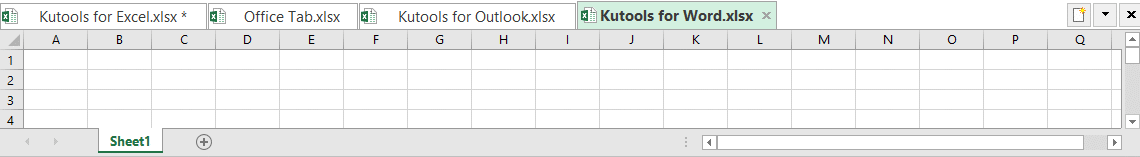0  产品特性